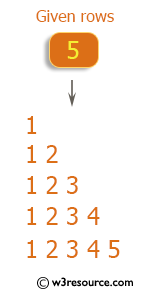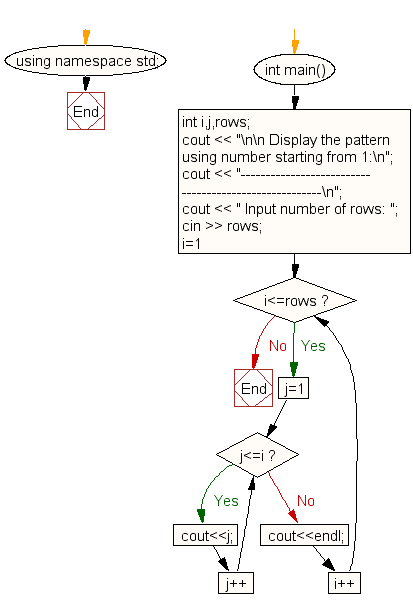﻿ C++ : Pattern like right angle triangle with number# C++ Exercises: Display the pattern like right angle triangle with number

## C++ For Loop: Exercise-36 with Solution

Write a program in C++ to display the pattern like right angle triangle with number.

Pictorial Presentation:Sample Solution:-

C++ Code :

``````#include <iostream>
using namespace std;

int main()
{
int i,j,rows;
cout << "\n\n Display the pattern using number starting from 1:\n";
cout << "------------------------------------------------------\n";
cout << " Input number of rows: ";
cin >> rows;
for(i=1;i<=rows;i++)
{
for(j=1;j<=i;j++)
cout<<j;
cout<<endl;
}
}
``````

Sample Output:

``` Display the pattern using number starting from 1:
------------------------------------------------------
Input number of rows: 5
1
12
123
1234
12345
```

Flowchart:C++ Code Editor:

Contribute your code and comments through Disqus.

What is the difficulty level of this exercise?

﻿